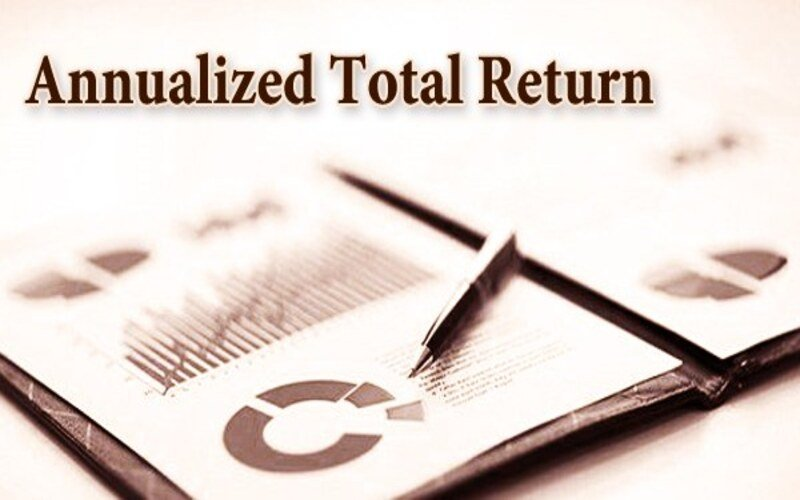Connect with us

Hi, what are you looking for?

DOGE0.070.84%SOL19.370.72%USDC1.000.01%BNB287.900.44%AVAX15.990.06%XLM0.080.37%

# Annualized Total Return Formula and CalculationPhoto: Annualized total return Photo: Annualized total return

How Does Annualized Total Return Work?

An investment’s geometric average yearly return over a specified period is the annualized total return. The annualized return formula uses a geometric average to compute what an investment would make over time if the yearly return were compounded.

Investors cannot determine an investment’s volatility or price swings from an annualized total return, simply offering a glimpse of its performance.

Knowing the annualized total return

We’ll examine the hypothetical performances of two mutual funds to understand annualized total returns. The annualized rate of return for the two funds during five years is provided below:

Returns on Mutual Fund A: 3%, 7%, 5%, 12%, and 1%
Returns from Mutual Fund B: 4%, 6%, 5%, 6%, and 6.7%

5.5% is the annualized rate of return for both mutual funds, although Mutual Fund A is substantially more unpredictable. While Mutual Fund B’s standard deviation is merely 1%, it has a standard deviation of 4.2%. Reviewing risk data is crucial, even when examining an investment’s annualized return.

Calculation & Formula for Annualized Return

Only two factors are required to determine the annualized rate of return: the length of time the investment was kept and the returns for a certain period. The equation is:

Annualized Return=((1+r1)×(1+r2)×(1+r3)××(1+rn))n11

For example, take the annual rates of returns of Mutual Fund A above. An analyst substitutes each ” r ” variable with the appropriate return and “n” with the years the investment was held. In this case, five years. The annualized return of Mutual Fund A is calculated as:

Annualized Return=((1+.03)×(1+.07)×(1+.05)×(1+.12)×(1+.01))511=1.3090.201=1.05531=.0553, or 5.53%

An annualized return does not have to be limited to yearly returns. Suppose an investor has a cumulative return for a given period. An annualized performance figure can be calculated even if it is a specific number of days. In that case, however, the annual return formula must be slightly adjusted to:

Annualized Return=(1+Cumulative Return)365Days Held−1

For example, assume a mutual fund was held by an investor for 575 days and earned a cumulative return of 23.74%. The annualized rate of return would be:

Annualized Return=(1+.2374)365575−1=1.145−1=.145,or 14.5%

Annualized Return vs. Average Return: Differences

Simple average calculations can only be performed when numbers are unrelated. The annualized return is employed because, as a result of compounding, the amount of investment lost or gained in one year is reliant on the amount from the prior years taken into account.

For instance, a mutual fund manager must achieve a 100% return to break even if she loses 50% of her client’s money. Comparing different mutual funds or the return of equities that have traded over multiple periods may be done more effectively using the more precise annualized return.

Annualized Return Reporting

Any investment that does not have a track record of at least 365 days cannot “ratchet up” its performance to be annualized by the Global Investment Performance Standards (GIPS), a set of regulated, industry-wide rules that direct the ethics of performance reporting.

As a result, if a fund has only been in operation for six months and has earned 5%, it is not permitted to claim that its annualized performance is about 10%. Doing so would be forecasting future performance rather than relaying facts from the past. In other words, determining an annualized rate of return requires using data from the past.

What Constitutes an Annualized Total Return?

The annualized total return measures an investment’s or a portfolio of investments’ typical yearly performance. Since it is calculated as a geometric average, the effects of compounding over time are considered. The compound annual growth rate (CAGR) is another name for annualized total return.

What Sets an Annualized Total Return Apart from an Average Return?

The annualized total return reflects the impacts of compounding, but the average return does not. This is the primary distinction between the annualized total return and the average return.

Consider the scenario of an investment with a 50% value loss in year one but a 100% return in year two. You would receive a 25% annual return by averaging these two percentages. Common sense, however, would suggest that the investor has evened out their investment (losing half its value in year one and recovering that loss in year two). The annualized total return, wero percent, would better reflect this truth.

What Is the Difference Between Compound Annual Growth Rate (CAGR) and Annualized Total Return?

Since both formulas aim to reflect the geometric return of an investment over time, the annualized total return and CAGR are theoretically equivalent. The primary distinction between them is that the annualized total return is often computed using returns from many years. Still, the CAGR is sometimes provided using only the starting and ending data. But this is more of a question of tradition. The two measurements are equivalent in content.

The geometric average annualized total return of an investment over a certain period is represented. By generating a geometric average, the annualized total return formula accommodates compounding when displaying the annual returns that the investment would produce during the holding period. Although the statistic offers a helpful overview of an investment’s success, it hides volatility and price swings.

Conclusion

• An investment’s geometric average yearly return over a specified period is the annualized total return.
• The annualized return formula calculates how much a shareholder would make over time if the yearly return were compounded.
• Only two factors are required to calculate the annualized rate of return: the length of time the investment was kept and the returns for a certain period.